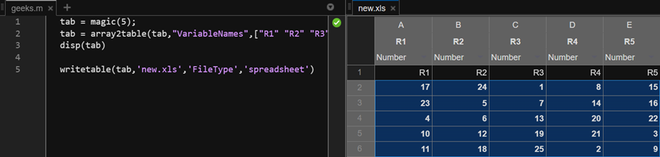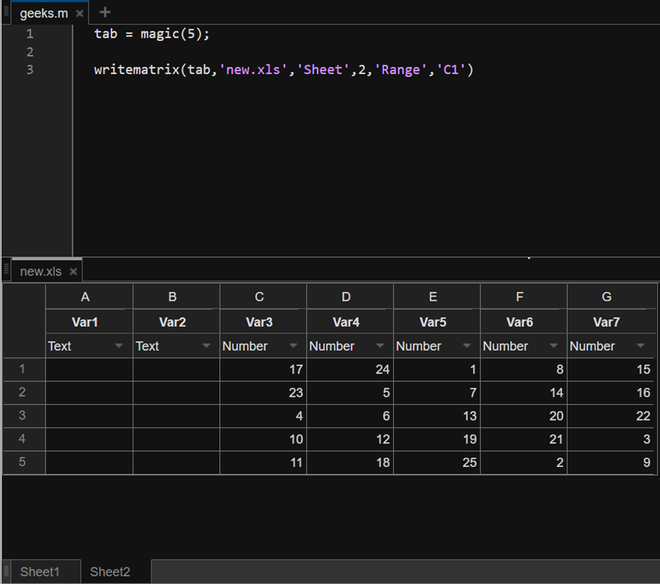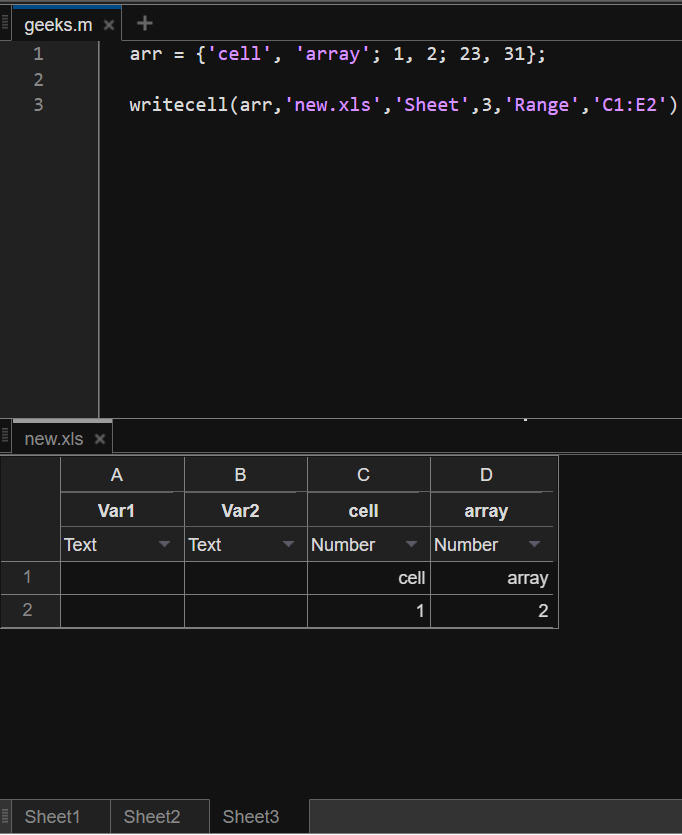GeeksforGeeks App
Open AppBrowser
Continue

# How to Write Data to Excel Spreadsheets in MATLAB?

MATLAB provides options to write a table, array, or matrix to Microsoft Excel spreadsheets. The function available to do so is the writetable () function. The general syntax for this function is:

Syntax:

writetable(<data>, <filename>, <optional_values>)

Now, in the following sections, we shall see how to write a table, an array, and a matrix into a spreadsheet.

## Writing a Table to Excel Spreadsheet:

Firstly, we shall create a table and then write the same to an excel spreadsheet with the help of writetable function.

Example 1:

## Matlab

 `% MATLAB Code``tab = magic(5);``tab = array2table(tab,``"VariableNames"``,``[``"R1"` `"R2"` `"R3"` `"R4"`  `"R5"``]);``disp(tab)`` ` `% Writing the table to excel file``writetable(tab,``'new.xls'``,``'FileType'``,``'spreadsheet'``)`

The output of the above code will create a new excel sheet in the current folder.In the above code, we create a table from magic with the variable names or table headers passed as a vector. Then, in the writetable function, we pass the table, and the file name to be used (if present then, it’ll overwrite the data. If not, then it will create a new file and then, it’ll create a new file). The next argument is a field type that decides the file type and the argument following it is the value for the same field; spreadsheet in this case.

## Writing a Matrix to Excel Spreadsheet

In the above section, we discussed how to add a table to an excel sheet. In this section, we shall explore the writetable further by adding a matrix at specified cells in a spreadsheet. Let us see the same with the help of an example.

To write numeric data into an excel sheet, we need can use the writetable function. We have to use another function, the writematrix function.

writematrix(<data>, <filename>, <optional_values>)

The syntax is the same as the writetable just the datatype changes to double, float, or int.

Example 2:

## Matlab

 `% MATLAB Code``tab = magic(5);`` ` `% Using the writematrix to write ``% numeric data at specific location.``writematrix(tab,``'new.xls'``,``'Sheet'``,2,``'Range'``,``'C1'``)`

Output:In this code, we write the magic square matrix to an excel spreadsheet named new.xls. The following arguments define the sheet number and the starting cell where we want to write our matrix-formed data.

## Writing a cell array (array of multiple data types) to an excel spreadsheet

To write an array with both numeric and text data, we use the writecell() function. The syntax of the same is similar to writematrix and writetable however, the data type then changes to a cell array.

writecell(<data>, <filename>, <optional_values>)

In the following example, we will write a cell array to a new sheet in our new.xls spreadsheet.

Example 3:

## Matlab

 `% MATLAB Code``arr = {``'cell'``, ``'array'``; 1, 2; 23, 31};`` ` `% writing cell array to a reduced range``writecell(arr,``'new.xls'``,``'Sheet'``,3,``'Range'``,``'C1:E2'``)`

Output:In this code, we are writing a 3×3 cell array to the spreadsheet in sheet 3 from a range of cells C1 to E2, this means that only as many elements as are specified in the range C1:E2.

My Personal Notes arrow_drop_up